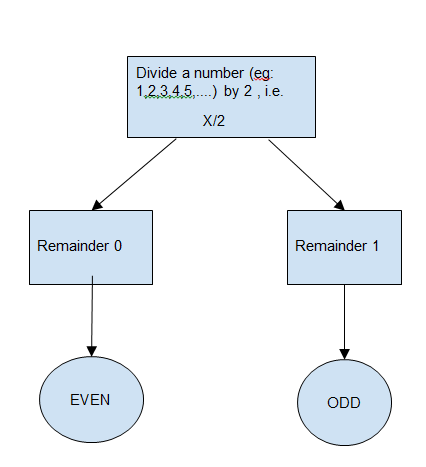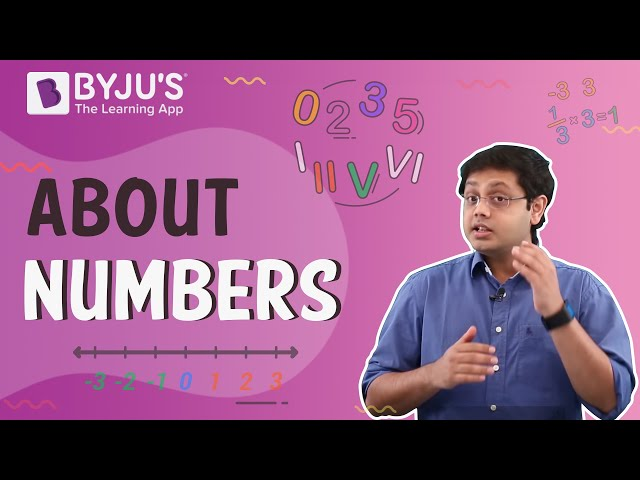Checkout JEE MAINS 2022 Question Paper Analysis : Checkout JEE MAINS 2022 Question Paper Analysis :

# Even And Odd Numbers

A number is an arithmetic value which is used to express the quantity of an object and helps in making calculations. The numbers are broadly classified into real numbers and imaginary numbers. In this article, we are going to discuss the sub-classification of real numbers called even and odd numbers in detail. Here, we will have a look at the definition, examples, and properties of even and odd numbers in detail.

## Definition of Even and Odd Numbers

Generally, the even and odd numbers are defined as follows:

Definition 1: “Even numbers are those numbers which are divisible by 2 and odd numbers which are not divisible by two”.

Definition 2: “Even numbers are those which when divided by 2 leaves no remainder or as 0 and Odd numbers are those numbers which when divided by 2 leaves a remainder of 1”.

## Set Representation of Even and Odd Numbers

The sets of even and odd numbers can be expressed as follows,

• Even = {2k : k
$$\begin{array}{l}\epsilon\end{array}$$
Z}
• Odd = {2k + 1 : k
$$\begin{array}{l}\epsilon\end{array}$$
Z}

A formal definition of an even number is an integer of the form n = 2k, where k is an integer. An odd number is defined as an integer of the form n = 2k + 1. This classification applies only to integers. Non-integer numbers like 1/2, 4.201, or infinity are neither even nor odd.

## Even and Odd Numbers Flowchart## Properties of Even and Odd Numbers

The following are the properties of even and odd numbers:

• The sum of two even numbers is an even number
• The sum of two odd numbers is an even number
• The sum of even and an odd number is an odd number
• Even number is divisible by 2, and leaves the remainder 0
• An odd number is not completely divisible by 2, and leaves the remainder 1.
• An even number ends with 0, 2, 4, 6, and 8
• An odd number ends with 1, 3, 5, 7, and 9

### How to Check a Number is an Even or Odd Number?

As we know now that “Even numbers those numbers which end with 0,2,4,6,8 and odd numbers are those numbers which end with 1,3,5,7,9.”

So, first, look at the number in the one’s place. This single number will tell whether the entire number is odd or even.

Let us take a number 1131. To check whether the given number is even or odd, first, divide the number by 2.

While dividing the number 1131 by 2, we get the remainder 1. So, the given number 1131 is an odd number.

## Video Lesson on Numbers## Even and Odd Numbers Examples

Some of the examples of even numbers are:

20, 46, 68, 100, 112, 446, and so on.

Some of the examples of odd numbers are:

21, 49, 67, 89, 111, 555, 999 and so on.

### What are Even and Odd Decimals?

Decimals are not even or odd numbers because they are not whole numbers. For example, you can’t say that the fraction 1/3 is odd by the fact that a denominator is an odd number or 12.34 as an even as its last digit is even. Only integers can be even, or odd, meaning decimals and fractions cannot be even or odd. Zero, however, is an integer and is divisible by two, so it is even. Odd numbers are those numbers not evenly divisible by two.

### Why Zero is an Even Number?

Zero is an even number because it is an integer multiple of 2, specifically 0 × 2.

Stay tuned with BYJU’S – The Learning App and download the app to learn with ease.

Quiz on Even and Odd Numbers